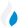•Trade Pi
•Trade
•Exchange
•Trade Pi
•Trade
•Exchange
\$1.1T
Total marketcap
\$64.82B
Total volume
40.14%
BTC dominance
•Trade Pi
•Trade
•Exchange

# SPIN to TRIVIA Exchange Rate - 1 Spintop in Trivians

0
• BTC 0.
Vol [24h]
\$0

## spin to trivia converter

Exchange Pair Price 24h volume
PancakeSwap (v2) SPIN/TRIVIA \$0.01358 \$0

## SPIN/TRIVIA Exchange Rate Overview

Name Ticker Price % 24h 24h high 24h low 24h volume
Spintop spin \$0.01262 0.7273% \$0.0127 \$0.01238 \$10.91K
Trivians trivia \$0.003474 -9.594% \$0.003886 \$0.00348 \$15.38K

Selling 1 SPIN you get 0 Trivians trivia.

Spintop Dec 24, 2021 had the highest price, at that time trading at its all-time high of \$0.6451.

408 days have passed since then, and now the price is 1.92% of the maximum.

Based on the table data, the SPIN vs TRIVIA exchange volume is \$0.

Using the calculator/converter on this page, you can make the necessary calculations with a pair of Spintop/Trivians.

## Q&A

### What is the current SPIN to TRIVIA exchange rate?

Right now, the SPIN/TRIVIA exchange rate is 0.

### What has been the Spintop to Trivians trading volume in the last 24 hours?

Relying on the table data, the Spintop to Trivians exchange volume is \$0.

### How can I calculate the amount of TRIVIA? / How do I convert my Spintop to Trivians?

You can calculate/convert TRIVIA from Spintop to Trivians converter. Also, you can select other currencies from the drop-down list.

## SPIN to TRIVIA Сonversion Table

SPIN TRIVIA
40 SPIN = 0 TRIVIA
90 SPIN = 0 TRIVIA
200 SPIN = 0 TRIVIA
300 SPIN = 0 TRIVIA
500 SPIN = 0 TRIVIA
900 SPIN = 0 TRIVIA
2000 SPIN = 0 TRIVIA
5000 SPIN = 0 TRIVIA
9000 SPIN = 0 TRIVIA
90000 SPIN = 0 TRIVIA
900000 SPIN = 0 TRIVIA
9000000 SPIN = 0 TRIVIA
90000000 SPIN = 0 TRIVIA